Start typing, then use the up and down arrows to select an option from the list.# The Electron Configuration

Jules Bruno
231views
1
so here. If we re imagine the periodic table will see in this depiction now realize here we have blue, We have yellow, We have purple, only have read sectors. Now each of these is called the bloc s. The S block is what's in blue. This is the P block, the D block and the F block. Remember, the S sub level has one orbital and that one orbital can hold a maximum of two electrons, Which is why the S block is these two columns to represent the two maximum electrons that the sub level could hold. The P sub level has three organs, right and each one again can hold a maximum of two electrons. So P theoretically can hold a maximum of six electrons. That's why the P block has 12345 and six slots for it. D can have up to 10 electrons because it has five orbital's, and if you count, you'll see in here there's 10 spots and then for the F sub levels, they can hold up to 14 electrons. And if you were to count these rows in red, you see, they come out to 14 now realize here that in this periodic table, the first slot here, which represents hydrogen, starts off our electron configuration as one s one. Then, as we move to the next one, we add another electron. So helium is one as to then when we get to the second row, since we're in the second row, we now have to. This is still the S block. So this is one s two to s one and it continues. Onward and onward. Over. Here we go. One asked to two s 22 p one. So, following this pattern, this would be three us for us five s, six s and seven s. And then here this would be three p four p five p six p and seven p. When we go to the D block, realize that it's gonna drop down by one. So here this is for us. But then when we go to D block, it drops down by one number. So now it's three D. This would be 40 five d and now notice how this number goes from 57 to 72. That's because 58 to 71 are here. Remember this this red line here says that this entire red row exist in between 57 72 and then we go between 104 because 90 to 103 is down here. These are your F blocks. They also dropped down by one number. So this is four F and five F. So, basically, if you can re imagine the periodic table in this fashion, you can use it to figure out the electron configuration of any elements or ion given to you. So we're gonna put this periodic table to use in order to do future electron configurations.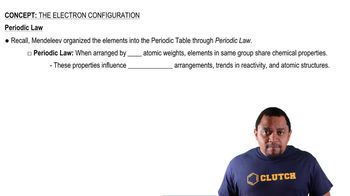00:41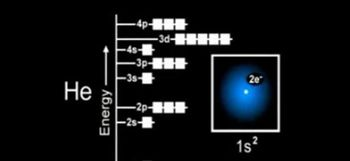01:49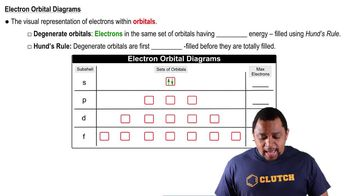02:15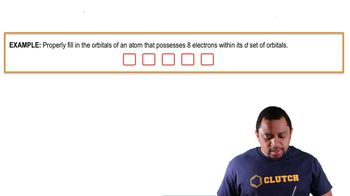00:50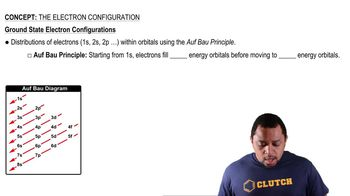01:32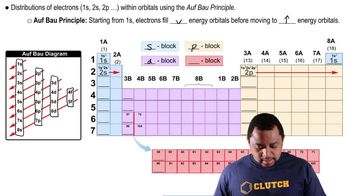03:04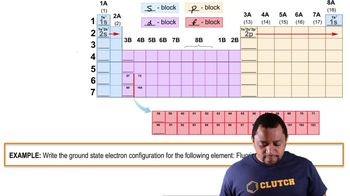01:33